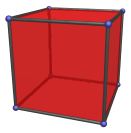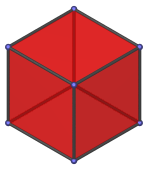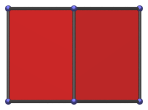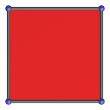# The Cube

The cube is one of the Platonic solids. It is bounded by 6 square faces, and has 8 vertices and 12 edges. It may be thought of as the 3D analogue of a square.The dual of the cube is the octahedron. The cube occurs as cells in the tesseract, as well as in various other 4D uniform polytopes. The full list is given below.

## Projections

In order to be able to identify the cube in various projections of 4D objects, it is useful to know how it appears from various viewpoints. The following are some of the viewpoints that are commonly encountered:

Projection Envelope DescriptionHexagon

Vertex-first parallel projection.Rectangle

Edge-first parallel projection. Two of the cube's faces project to the top and bottom edges of the projection envelope.Square

Face-first parallel projection. Four faces project to the edges of the projection envelope.

## Coordinates

The Cartesian coordinates of a cube centered on the origin with edge length 2 are:

• (±1, ±1, ±1)

## Occurrences

The cube occurs in the following 4D polytopes:

Last updated 10 Jan 2019.